##Vecten Configuration

On the sides of triangle t=(DEF) erect squares.
The figure has may interesting properties. Among them the simplest shown below. The triangles DEI and LEF are equal. DI and LF intersect orthogonally. Line EG passes through the center H of square DFMN.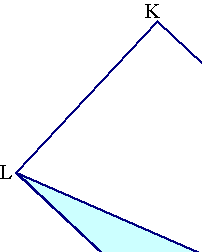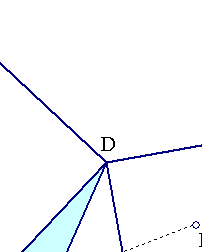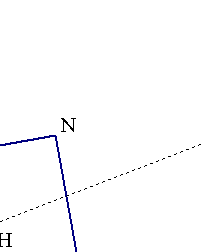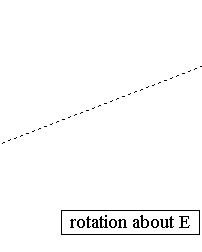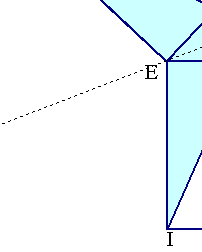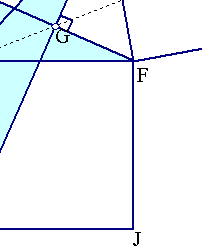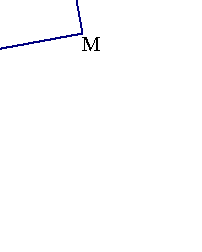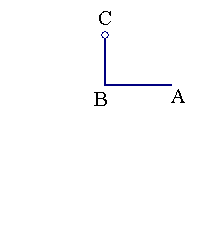Triangle LEF can be rotated about E. The angle of rotation is <(ABC). You can catch and modify the location of C and see the rotation. Further study of the subject is contained in the file: Vecten2.html .

 Produced with EucliDraw© http://users.math.uoc.gr/~pamfilos/